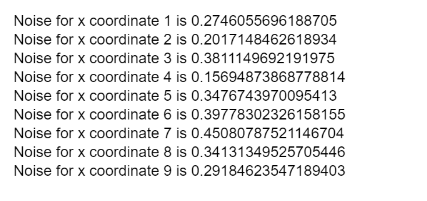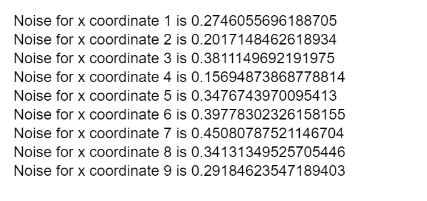# p5.js | noiseSeed() Function

• Last Updated : 29 Jan, 2020

The noiseSeed() function is used to set a seed value for the noise() function. The noise() function, by default returns a number that is semi-random, meaning that the noise value would be the same for a coordinate only until the program is running. Running the program for the next time would yield a different value.

These noise values can be made constant by setting a non-changing seed value in the program so that it returns the same values every time when the program is run.

Syntax:

`noiseSeed( seed )`

Parameters: This function accepts a single parameter as mentioned above and described below:

• seed: This parameter holds a number which represents the seed value.

Return Value: This function does not return any value.

Below example illustrates the noiseSeed() function in p5.js:

Example:

 `let x_coordinate = 0.0;``let plot_y = 0.0;``  ` `function` `setup() {``    ``createCanvas(400, 200);``}``  ` `function` `draw() {``  ` `    ``// Specifying a noise seed value``    ``noiseSeed(100);``  ` `    ``if` `(x_coordinate < 10) {``         ` `        ``// Get noise with x coordinate``        ``x_noise = noise(x_coordinate);``    ` `        ``// Output the noise along with``        ``// its corresponding coordinate``        ``coord_text = ``"Noise for x coordinate "``            ``+ x_coordinate + ``" is "` `+ x_noise;``         ` `        ``text(coord_text, 10, plot_y);``  ` `        ``// Increment the x coordinate``        ``x_coordinate++;``  ` `        ``// Increase the y coordinate``        ``// for plotting``        ``plot_y = plot_y + 15;``    ``}``}`

Output: The values are constant every time the program is run.

• Running the program for the first time:• Running the program for the second time:Online editor: https://editor.p5js.org/
Environment Setup: https://www.geeksforgeeks.org/p5-js-soundfile-object-installation-and-methods/

My Personal Notes arrow_drop_up